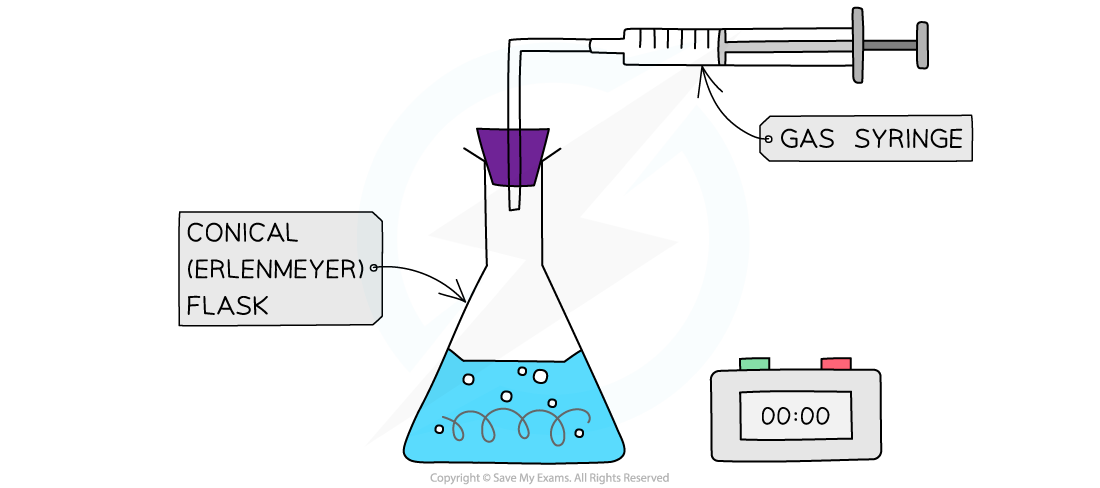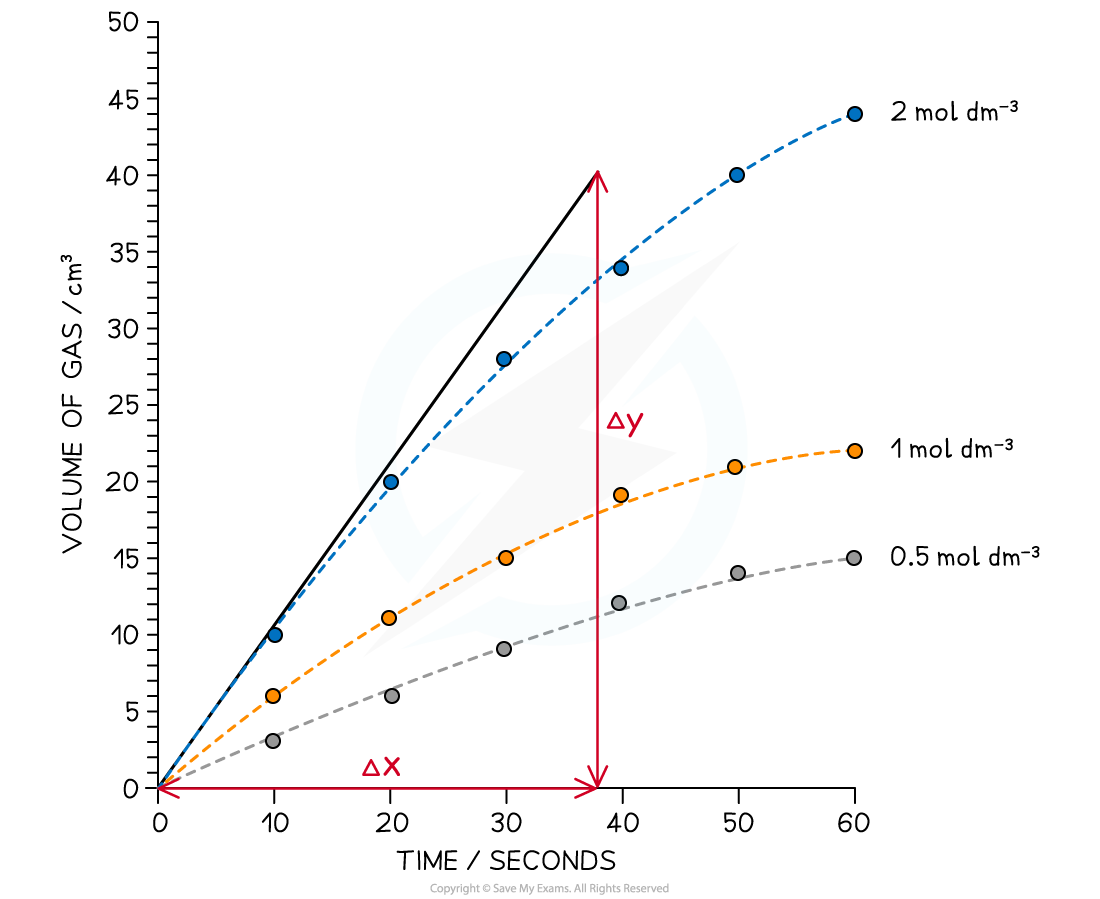# AQA A Level Chemistry复习笔记8.1.1 Required Practical 7

### Required Practical 7

#### Required Practical 7: Measuring the rate of a reaction

• There are two ways to measure the rate of reaction
• By an initial rate method
• By a continuous monitoring method

An initial rate method: The reaction between magnesium and hydrochloric acid

• This reaction can be used to investigate the effect of varying the concentration of the acid while keeping the temperature constant
• When a gas is released in a reaction you can either try to measure the volume of gas given off or the mass change in the reaction flask
• However, in this case the gas hydrogen is too low in density so the mass change will be far too small to register on a laboratory balance

• Volume can be measured either by displacement of water into an inverted measuring cylinder or by using a gas syringeThe rate of reaction between magnesium and dilute hydrochloric acid can be measured using a gas syringe and stopwatch

Steps in the procedure

• Before you begin you need to check your apparatus is gas tight
• This can be done by assembling everything without the acid or magnesium and trying to move the plunger
• If you feel resistance the apparatus is gas tight

• The acid will go in first because it is quicker to drop a piece of magnesium ribbon in than to pour in the acid
• To vary the concentration of the acid you need to dilute it by measuring portions of acid in a measuring cylinder and then portions of distilled water in another measuring cylinder and adding them to the conical flask
• Choose a suitable volume of acid to match the size of the flask, e.g. 40 cm3
• You don't want to use acid that is stronger than 2 mol dm-3 because the reaction will be too fast, so its best to start with 2 mol dm-3 and perform a serial dilution, e.g. 40 cm3(acid) + 0 cm3 (water), 35 cm3 (acid) + 5 cm3 (water), etc

Practical tips

• Make sure the plunger is fully inserted before you start the experiment otherwise you will have a volume error
• If the magnesium does not look new and shiny, you may need to clean the surface with a bit of sandpaper
• Make sure the plunger is secure and does not fall out of the barrel if the volume exceeds 100 cm3

Specimen Results

• Here is a set of typical results for this experiment

Rate of reaction between magnesium and acid results tableGraphing the resultsGraph showing typical results for the rate of reaction between hydrochloric acid and magnesium at different concentrations

Analysis

• The lines of best fit are drawn for each concentration on the same graph
• A tangent is then drawn starting from (0,0) since this method is to find the initial rate of reaction
• The gradient of the tangent is determined which gives the rate of reaction
• In the example above, the rate of reaction for 2.0 mol dm-3 acid is